Question

Write the equation of the line that passes through the points (0, -3) and (-4,1).
Put your answer in fully reduced point-slope form, unless it is a vertical or horizontal
line.

1.ngockhue

y=-1x-3

Step-by-step explanation:

First, you have to find the slope using the formula I attached. Using this formula, you will subtract the second y from the first y, which is 1-(-3) for this problem. That equals 4, so that will be the numerator of the slope. Next, you have to subtract the second x from the first x, which is -4-0 for this problem. That equals -4, so that will be the denominator for this slope. the fraction now is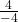, which is -1. The slope is -1. The current equation looks like this: y=-1x+b. Now we can solve for b, or the y-intercept. To do this, you have to use the x and y of one of the coordinates, it doesn’t matter which one, the answer will be the same. Use the first one, for example. The x is 0 and the y is -3, so the our equation to find b is -3=-1(0)+b, which is -3=0+b, which is -3=b, or b=-3. So now we know what b is. Now we have to two components we need to write the equation, the slope (-1) and the y-intercept (-3). Now we can write the equation. y=-1x+(-3), or y=-1x-3.

2.Step-by-step explanation:

I’m going to explain this in standard slope intercept form (y=mx+b) because it is easiest for this situation. I will also include converting it to point slope form. I will also show how to do straight into point slope form if you find that more helpful.

First thing is to identify difference in x and y in both points. From point 1 to point to, the x changes -4 and the y changes +4. We can rapresent this in rise over run fraction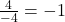So the slope is -1 because the slope is the simplified rise over run fraction.

Our next step is to find the y-intercept. This is easy because the first point is the y-intercept, so -3 is our y-intercept.

Now we know the equation.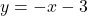All we have to do now is convert it into point-slope form. So here is point slope form: y + y1 = m(x + x1). Y1 is the y-intercept and m is the slope.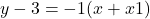So x1 is the x intercept, so to find this you find the x value when y is 0. You can do this by using the standard form I’ve done because it is easiest.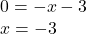So when y is 0, then x is -3. Here is the complete point slope form of the line.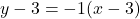—————————————————–

To put the line directly into point slope for you need to find the slope, y-intercept, and x-intercept and place the values in accordingly. If you aren’t given the y-intercept and x-intercept straight away it can be difficult for some putting it directly in point slope form. That is why I recommend putting it into standard form then point-slope form.